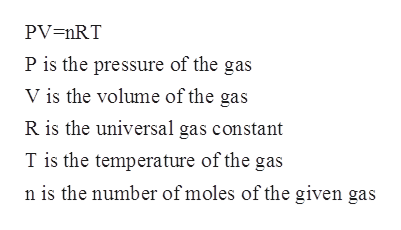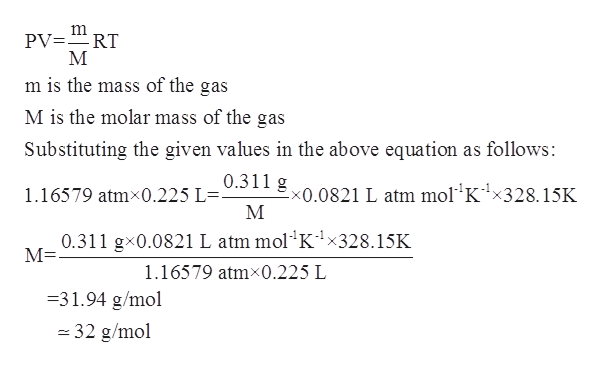# A sample of a gas has a mass of 0.311f. At 55C, its volume is 0.225L & its pressure is 886 mmHg. Find its molar mass.

Question
4 views
A sample of a gas has a mass of 0.311f. At 55C, its volume is 0.225L & its pressure is 886 mmHg. Find its molar mass.
check_circle

Step 1

The number of moles for a given chemical species refers to the ratio of mass of the species to that of its molar mass. The formula is shown below:

Step 2

The ideal gas equation is known as the general gas equation and is a equation of state for a given hypothetical gas. The equation is given by:help_outlineImage TranscriptionclosePV=nRT P is the pressure of the gas V is the volume of the gas R is the universal gas constant T is the temperature of the gas n is the number of moles of the given gas fullscreen
Step 3

In the given problem, the temperature is given to be 55oC =328.15 K.

The pressure of the gas is given to be 886 mmHg=1.16579 atm.

Volume occupied by the gas is given to be 0.225 L...help_outlineImage TranscriptionclosePV RT m is the mass of the gas M is the molar mass of the gas Substituting the given values in the above equation as follows: 0.311 g x0.0821 L atm mol K1x328.15K 1.16579 atmx0.225 L=- М 0.311 gx0.0821 L atm mol K1x328.15K М- 1.16579 atmx0.225 L 31.94 g/mol 32 g/mol fullscreen

### Want to see the full answer?

See Solution

#### Want to see this answer and more?

Solutions are written by subject experts who are available 24/7. Questions are typically answered within 1 hour.*

See Solution
*Response times may vary by subject and question.
Tagged in

### Physical Chemistry